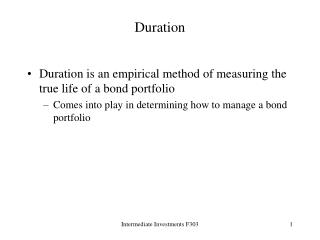DownloadDownload PresentationDuration

# Duration

Télécharger la présentation## Duration

- - - - - - - - - - - - - - - - - - - - - - - - - - - E N D - - - - - - - - - - - - - - - - - - - - - - - - - - -
##### Presentation Transcript

1. Duration • Duration is an empirical method of measuring the true life of a bond portfolio • Comes into play in determining how to manage a bond portfolio Intermediate Investments F303

2. Six Propositions Regarding Interest Rate Sensitivity • Bond prices and yields are inversely related • An increase in the yield to maturity results in a smaller price decline than the price gain associated with a decrease of equal magnitude • Prices of long-term bonds tend to be more sensitive to interest rate changes than prices of short-term bonds • The sensitivity of bond prices to changes in yield increases at a decreasing rate as maturity increases • Interest rate risk is inversely related to the bond’s coupon rate. So, prices of high coupon bonds are less sensitive to changes in interest rates than prices of low coupon bonds • The sensitivity of a bond’s price to a change in its yield is inversely related to the yield to maturity at which the bond is currently selling Intermediate Investments F303

3. Conclusions on Interest Rate Sensitivity? • Maturity of a bond is a major determinant of interest rate risk • Consequently, the concept of Duration, the actual term of a bond portfolio, is critical to assessing the riskiness of a bond portfolio • Higher coupon bonds are less price sensitive than low coupon bonds • And Zero coupon bonds are consequently the most sensitive of all! • Zero coupon bonds are thus also considered a longer-term investment Intermediate Investments F303

4. Duration Redefined • Duration is a measure of effective maturity of a bond, defined as the weighted average of the maturities of each payment with weights proportional to the present value of the payment Intermediate Investments F303

5. What is the Duration of a 6% coupon, 5 year bond yielding 8%? Intermediate Investments F303

6. What is the Duration of a 10% coupon, 5 year bond yielding 8%? Intermediate Investments F303

7. Another Exercise • What is the interest rate sensitivity of the following two bonds? Assume coupons are paid annually Bond ABond B Coupon rate 10% 0% Face value \$1,000 \$1,000 Maturity 5 years 10 years YTM 10% 10% Price \$1,000 \$ 385.54 Intermediate Investments F303

8. Exercise (cont) Intermediate Investments F303

9. Modified Duration • Practitioners actually use a concept called Modified Duration • Modified Duration = Duration / (1 + Yield) • You can use Modified Duration to project the new price of a bond whenever there is a change in yield • The change in price = (- modified duration * change in yield) * previous price Intermediate Investments F303

10. Modified Duration (cont) • Using the two bonds in our example, what would be the change in price of the bonds if the yield increased from 10% to 10.1%? Conclusion: In order to analyze bond volatility, duration and not maturity is the appropriate measure Intermediate Investments F303

11. How Accurate Are Duration Calculations? • Consider the two five year bonds from our first example, but assume interest rates change by moving 3% up or down: • What is the price change using modified duration? • Use the table below to find the actual price change: • Note: Average change based on absolute values Intermediate Investments F303

12. How Accurate Are Duration Calculations? • Modified duration of bonds yielding 8% • Bond A 6% coupon, duration 4.44 years: 4.44/1.08 = 4.11 • Bond B 10% coupon, duration 4.20 years: 4.20/1.08 = 3.89 • So: • .03 * 4.11 = .1233 or 12.33% v. actual average of 12.39% • .03 * 3.89 = .1167 or 11.67% v. actual average of 11.73% • If you compare to actual not average changes • Understate positive changes and overstate negative changes Intermediate Investments F303

13. Conclusions Regarding Duration • Using modified duration to predict changes in price v. actual changes becomes imprecise for large interest rate movements • Nonetheless, Duration is a key to managing fixed income security portfolios for three reasons: • It is an effective measure of the average maturity of a portfolio • It provides a tool for immunizing portfolios from interest rate risk • It is a measure of interest rate sensitivity of a bond portfolio Intermediate Investments F303

14. Five Rules Regarding Duration • The duration of zero coupon bonds equals their time to maturity • Holding time and yield to maturity constant, a bond’s duration and interest rate sensitivity are higher when the coupon rate is lower • Holding the coupon rate constant a bond’s duration and interest rate sensitivity generally increase with time to maturity • Holding other factors constant, the duration and interest rate sensitivity of a coupon bond are higher when the yield to maturity is lower • The duration of a level perpetuity is equal to (1 + Yield) / Yield Intermediate Investments F303